Go to content
2 marks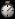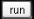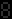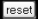This display is based on a typical examination question. You can change some of the details in the question and then see the answer, step by step.

A similar question appeared on the GCSE Foundation exam in 2003.

Summary/Background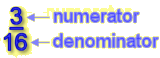The top part of a fraction is called the numerator; the bottom part is called the denominator. You need to know how to simplify, add, subtract, multiply and divide fractions, and how to use the "Fractions" button on your PAGE(calculator,calculator).You can get a better display of the maths by downloading special TeX fonts from jsMath. In the meantime, we will do the best we can with the fonts you have, but it may not be pretty and some equations may not be rendered correctly.

Glossary

denominator

the integer on the bottom of a fraction

fraction

A ratio of two numbers or polynomials.

numerator

the integer on the top of a fraction

union

The union of two sets A and B is the set containing all the elements of A and B.

Full Glossary List

This question appears in the following syllabi:

SyllabusModuleSectionTopicExam Year
AQA GCSE (9-1) Foundation (UK)N: Structure and CalculationN8: Exact CalculationsCalculations with Fractions-
CBSE IX (India)Number SystemsReal NumbersNumber types-
CIE IGCSE (9-1) Maths (0626 UK)1 NumberB1.5 Decimals, Fractions and PercentagesUnderstanding Fractions-
Edexcel GCSE (9-1) Foundation (UK)N: Structure and CalculationN8: Exact CalculationsCalculations with Fractions-
GCSE Foundation (UK)NumberFractionsUnderstanding fractions2003
OCR GCSE (9-1) Foundation (UK)2: Fractions, Decimals and Percentages2.01b: Calculations with FractionsFurther Fractions-
Universal (all site questions)FFractionsUnderstanding fractions-